# Catch up on the latest AI articles# Model Compression For Unconditional-GAN

3 main points
✔️ Model compression techniques for Unconditional-GANs
✔️ Proposes a pruning and knowledge distillation that emphasizes the retention of important content in images
✔️ Equal or better performance compared to existing compression techniques and original models

written by Yuchen LiuZhixin ShuYijun LiZhe LinFederico PerazziS.Y. Kung
(Submitted on 6 Apr 2021)

Subjects: Computer Vision and Pattern Recognition (cs.CV)code：The images used in this article are from the paper, the introductory slides, or were created based on them.

## first of all

Generative Adversarial Network (GAN) has shown excellent results in tasks such as image generation and image editing.

However, state-of-the-art GANs, such as StyleGAN2, face challenges in terms of computational complexity and memory cost, making it difficult to deploy them on edge devices.

However, these methods cannot be directly applied to Unconditional-GANs such as StyleGAN. In this article, we propose a model compression method specialized for Unconditional-GAN to deal with this problem. Let's take a look at it below.

## proposed method

The goal of the proposed method is to obtain a smaller capacity and more efficient model $G'$ for $G$, an Unconditional-GAN that generates images from random noise.

More specifically, the goal is to achieve the following two things

1. For the same latent variable $z \in \mathcal{Z}$, the visual quality of the generated images $G(z), G'(z)$ is similar
2. For a real-world image $I \in \mathcal{I}$, the corresponding latent variables $Proj(G,I),Proj(G',I)$ are similar

The following Channel Pruning and Knowledge Distillation will be conducted to achieve this goal.

### Content-Aware Model Compression

Before we get into the details of Channel Pruning and Knowledge Distillation, let's consider a case where we want to compress a GAN that produces an image of a human face. In this case, the most important region of the generated image is the human face (other background regions are not important).

Therefore, in the original paper, we use a network (content-parsing neural network) that identifies regions (e.g., human faces) that correspond to important content in the images generated by the GAN. For Channel Pruning, we use a metric ($CA-l1-out$) that measures "how much each channel contributes to the generation of important content in the image". For Knowledge Distillation, we use a loss defined as the inheritance of "knowledge for the generation of important content in the image".

By using such "Content-Aware" model compression, we aim to obtain a lightweight model with the same quality as the original GAN.

The pruning of the proposed method measures the importance of a channel based on the following pipeline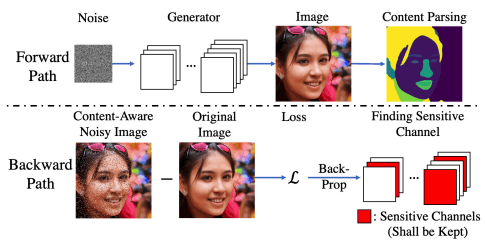The pipeline consists of two paths: Forward path/Backward path.

First, in the Forward path, the latent variable $z \in \mathcal{Z}$ is used to find the generated image $G(z) \in R^{H×W×3}$.

Next, we pass the generated image through $Net_p$, a network that predicts the content mask $m \in R^{H×W}, m_{h,w} \in {0,1}$ in the image (content-parsing neural network), and obtain the content map $COI={(h, w)|m_{h,w}=1}$.

In the generation of human face images, the human face region corresponds to this.

Next, in the Backward path, we prepare an image $G_N(z)$ with random noise only on the COI, which is an important region of the generated image $G(z)$. This is the Content-Aware Noisy Image in the above figure. For example, in the face image generation, the noise is added only to the human face region in the generated image.

Then, we perform back-propagation of the differentiable loss $L_{CA}(G(z),G_N(z))$ obtained from the original image to obtain the gradient $\nabla g\in R^{n_{in}×n_{out}×h×w}$.

By doing this for several random noise samples $z$, we obtain the expected value of the gradient $E[\nabla g]$. The importance measure $CA-l1-out$ for each channel $C_i$ is defined as the L1-norm of the gradient output for each channel.

$CA-l1-out(C_i)=||E[\nabla g]_i||_1, E[\nabla g]_i \in R^{n_{out}×h×w}$.

During the pruning process, the channels with this $CA-l1-out$ larger are retained, and the channels with this $CA-l1-out$ smaller are deleted.

In the proposed method Knowledge Distillation, we use a combination of several losses. Let's look at each of them in turn.

Pixel-level Distillation

The simplest example would be a loss defined to reduce the norm between the output or intermediate layers of the generated image $G(z), G'(z)$.

This is represented by the following equations for the output and intermediate layers, respectively

$L^{norm}_{KD}=E_{z \in \mathcal{Z}}[||G(z),G'(z)||1]$(output only)

$L^{norm}_{KD}=\sum^T_{t=1}E_{z \in \mathcal{Z}}[||G_t(z),f_t(G'_t(z))||1]$(middle layer)

where $G_t(z),G'_t(z)$ is the activation of the middle layer ($t$th layer) and $f_t$ is the linear transformation to match the depth dimension.

Image level Distillation

Apart from the pixel-level norm between the output images, we can also use an evaluation metric that more closely resembles the human judgment of similarity.

The proposed method uses the perceptual distance between the output images by LPIPS.

$L^{per}_{KD}=E_{z \in \mathcal{Z}[LPIPS(G(z),G'(z))]}$

Content-Aware Distillation

As in the case of pruning, the proposed method uses a distillation loss that focuses on the important content in the image. This is shown in the following figure.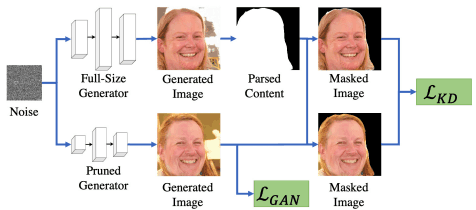Simply put, we compute the aforementioned pixel-level and image-level Distillation loss for the image after masking non-essential content regions in the image (e.g., background regions in face image generation).

By using such Content-Aware (focusing on the important content among the generated images) distillation losses, we can inherit more details of the important content among the generated images of the original GAN. By combining these distillation losses with the learning loss $L_{GAN}$ of the regular GAN, the final learning goal is as follows.

$L=L_{GAN}+\lambda L^{norm}_{KD} + \gamma L^{per}_{KD}$

When training the student model, we first derive the student generator $G'$ by pruning the generator $G$ of the teacher GAN, and the student Discriminator $D'$ uses the teacher Discriminator as it is. We then fine-tune both $G', D'$ by standard min-max optimization.

## experimental results

In our experiments, we performed model compression for SN-GAN trained on CIFAR-10 and StyleGAN2 trained on FFHQ. The resolution of the output image is 32px for SN-GAN and 256,1024px square for StyleGAN2.

To evaluate the performance of the generated images, we use the following five quantitative metrics

• Inception Score(IS): A measure of the classification quality of the generated images.
• Frechet Inception Distance (FID ): a measure of the similarity between the generated image and the real image.
• Perceptual Path Length(PPL): a measure of the smoothness of the latent space of a GAN.
• PSNR/LPIPS: Measures the similarity between a real image and an image generated from the latent vector by converting the image into a latent vector using GAN Inversion.

The last evaluation index for PSNR and LPIPS is shown in the following figure.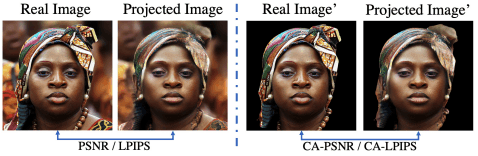In GAN-based image editing methods (e.g., this study ), such a metric is introduced because it is important to obtain the latent vector corresponding to the real image and to be able to recover the original image from it.

In addition, we also measure the similarity (CAxPSNR/CA-LPIPS in the above figure) between images in which the regions other than the important content in the image are masked, because these image editing methods, etc. place importance on whether the important content in the image is retained.

### About the proposed method (channel pruning)

At the outset, the results of the pruning of the proposed method and its comparison with various baselines are as follows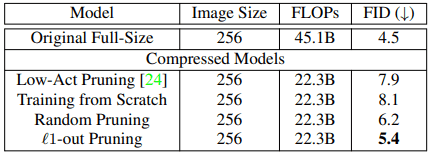Low-Act pruning, an existing pruning method, nearly fails at model compression for GANs, performing worse than random pruning and nearly as well as models trained from scratch.

On the other hand, the proposed pruning method resulted in only a 0.9 reduction in FID score while keeping FLOPs to half of the original.

### About the proposed method (knowledge distillation)

For the aforementioned learning goal $L=L_{GAN}+\lambda L^{norm}_{KD} + \gamma L^{per}_{KD}$ in knowledge distillation, the results of experiments with various settings of hyperparameters such as $\lambda and \gamma$ are as follows ( The trained model has 80% of the channels of the original model removed by the proposed pruning method).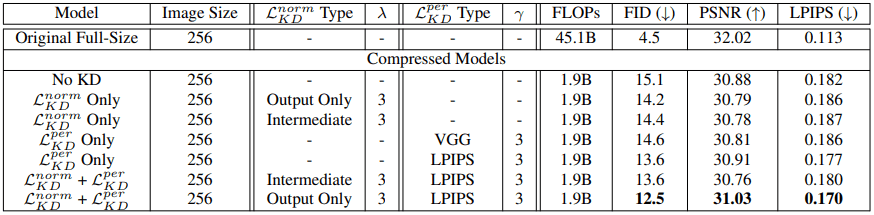In general, the best results were obtained when training was performed by $L^{norm}_{KD}$ with output only and $L^{per}_{KD}$ with LPIPS.

### Comparison with state-of-the-art GAN compression methods

Furthermore, we perform a comparison experiment between the proposed method and GAN Slimming (GS), a state-of-the-art GAN compression method. The results are as follows.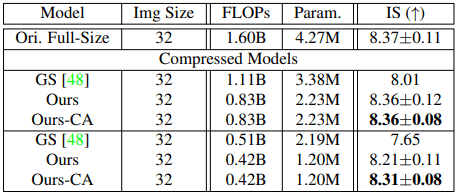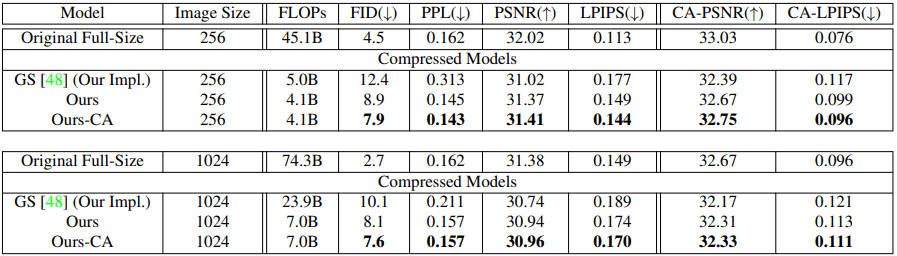In general, for both CIFAR-10/FFHQ, the proposed method (especially in the Content-Aware setting) showed better results.